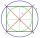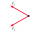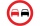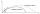# Fundamentals of Physics + vector - math problems

#### Number of problems found: 8

• Angle ASBOn a circle with a radius of 10 cm and with a center S, the points A, B, C are given so that the central angle ASB is 60 degrees and the central angle ASC is 90 degrees. Find the length of the circle arc and the amount of AB and AC offsets.
• Mass pointTwo equal forces of 30 Newtons act on a mass point. Find the magnitude of the resultant force if these forces form an angle of 42°.
• Angled cyclist turnThe cyclist passes through a curve with a radius of 20 m at 25 km/h. How much angle does it have to bend from the vertical inward to the turn?
• OvertakingOn the direct road, the passenger car overtakes the slower bus by starting to overtake 20 meters from the bus and after passing it ahead of it again 20 meters away. The car overtakes at a steady speed of 72 km/h, the bus goes at a steady speed of 54 km/h.
• ParatrooperAfter the parachute is opened, the paratrooper drops to the ground at a constant speed of 2 m/s, with the sidewinding at a steady speed of 1.5 m/s. Find: a) the magnitude of its resulting velocity concerning the ground, b) the distance of his land from a
• Forces on earth directionsA force of 60 N [North] and 80 N [East] is exerted on an object wigth 10 kg. What is the acceleration of the object?
• Ballistic curveThe soldier fired the ballistic grenade at a 45° angle. The first half ascended, the second fall. How far and height it reached if his average speed was 1200 km/h, and 12 seconds took from the shot to impact.
• Two forcesTwo forces with magnitudes of 25 and 30 pounds act on an object at 10° and 100° angles. Find the direction and magnitude of the resultant force. Round to two decimal places in all intermediate steps and your final answer.

We apologize, but in this category are not a lot of examples.
Do you have an exciting math question or word problem that you can't solve? Ask a question or post a math problem, and we can try to solve it.

We will send a solution to your e-mail address. Solved examples are also published here. Please enter the e-mail correctly and check whether you don't have a full mailbox.

Our vector sum calculator can add two vectors given by their magnitudes and by included angle. Fundamentals of Physics - math problems. Vector - math problems.